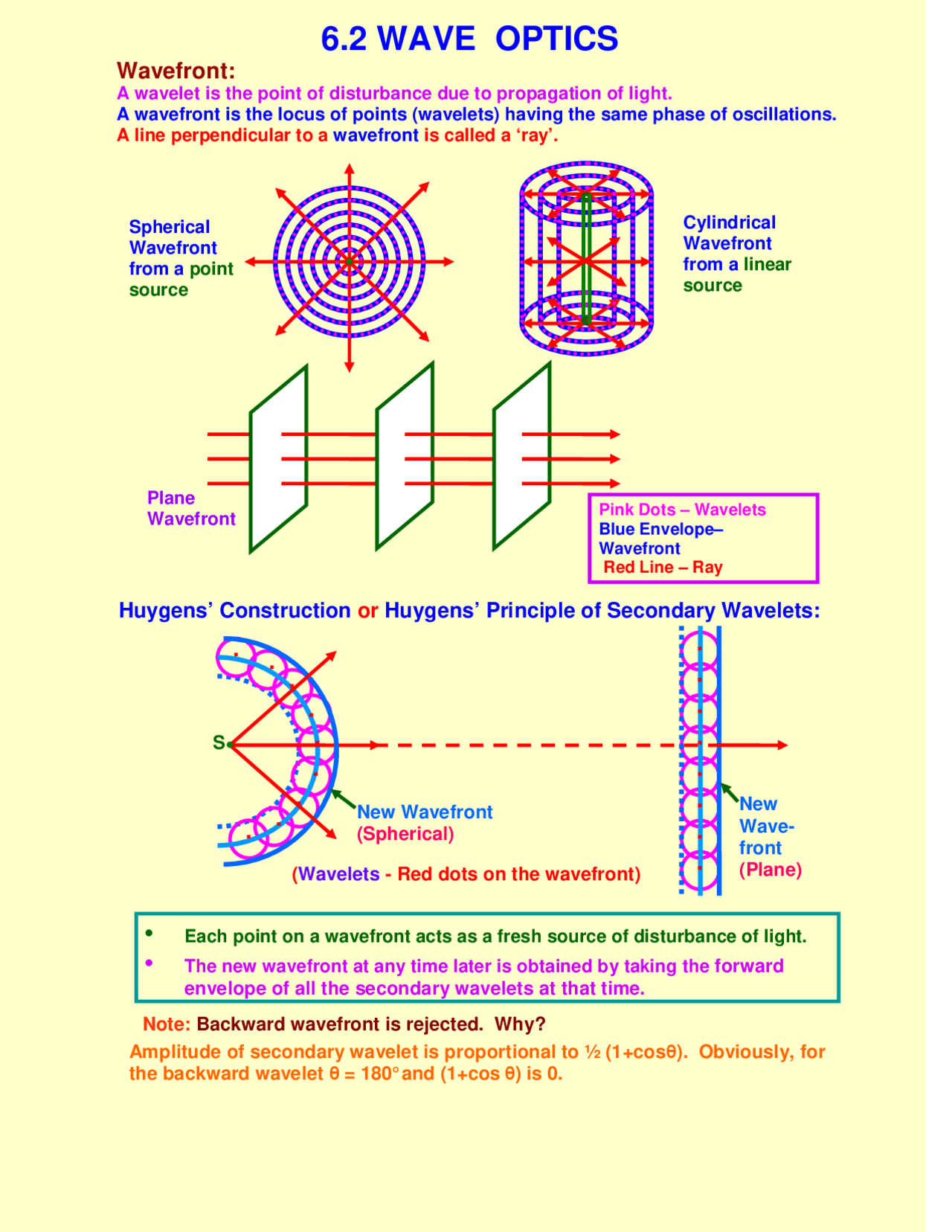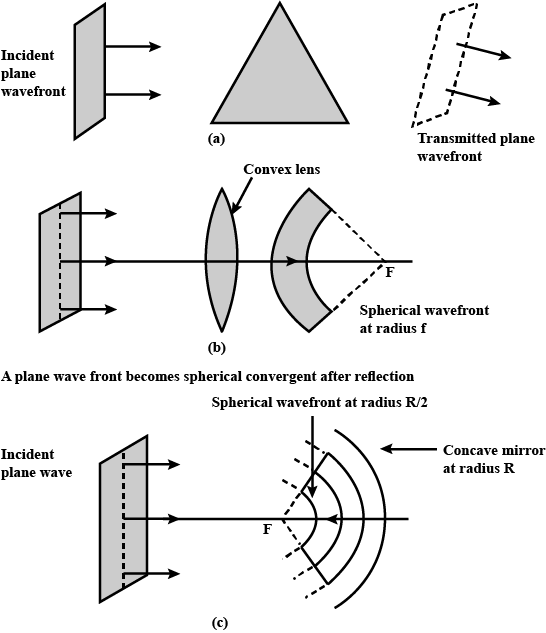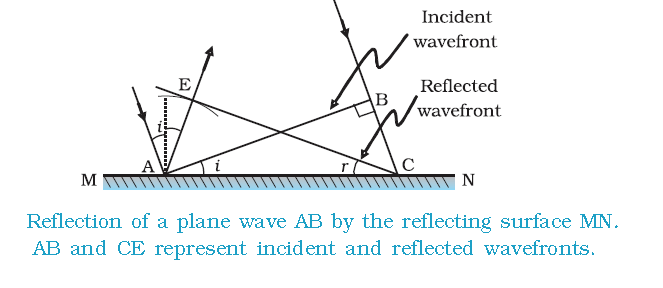# CBSE Class 12 Physics Chapter 10 Revision Notes

Chapter 10: Wave Optics Revision Notes

• Wave Optics: describes the relationship between waves and light rays. Light is a form of energy that travels through a medium as a transverse wave, according to the wave theory of light.Source

• Wave Front: The wave front is the location of all particles vibrating in the same phase at any given time. As a result, a wave front is a surface that has the same phase of vibrating particles at every point on it at any given time.
• Phase Speed: Wave speed is equal to phase speed, which is the rate at which the wave front moves.
• A point source has a spherical wavefront, a line source has a cylindrical wavefront, and a source at infinity has a plane wavefront.
• A ray is a line that runs perpendicular to a wave front. Rays are always perpendicular to the wave front as they travel along the wave’s propagation path.
• Huygens’ principle is essentially a geometrical construction that allows us to determine the shape of the wave front at any time. Huygens’ principle states that

(i) Every point on a wave front emits secondary wavelets and behaves like a light source.

(ii) With the speed of light, secondary wavelets spread in all directions in space (vacuum).

(iii) The new position of the wave front is determined by the envelope of secondary wavelets along the forward direction after a given time.

• Huygens’ wave theory can be used to verify the laws of reflection and refraction.
• Huygens’ wave theory successfully explains interference, diffraction, and polarization phenomena.
• Since frequency v is a source characteristic, v = 1/T remains constant as light travels from one medium to another.
• Wavelength is inversely proportional to the medium’s refractive index (μ), i.e. λ’ = λ/μ

## Behaviour of a Prism, Lens, and Spherical Mirror Towards Plane Wave FrontSource

## Reflection laws based on Huygens’ theory of waves

• Consider a plane wave front AB incident on a reflecting surface XY, with both the wave front and the reflecting surface perpendicular to the plane of paper, as shown in the diagram.Source

]]>